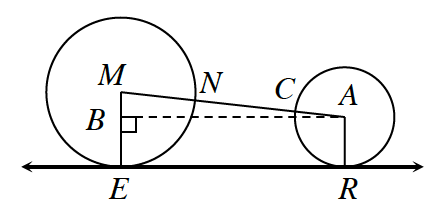### Home > INT2 > Chapter 10 > Lesson 10.2.4 > Problem10-94

10-94.In the diagram at right, $⊙M$ has a radius of $14$ feet and $⊙A$ has a radius of $8$ feet. $\overleftrightarrow { E R }$ is tangent to both $⊙M$ and $⊙A$. If $NC = 17$ feet, what is $ER$?

Use the given information to find the length of the hypotenuse of $ΔMBA$.

How can you use the radius of each circle to determine $MB$?

Notice that the length of the dotted line is equivalent to $ER$.

$≈ 38.5 \; \text{feet}$